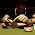## Pages

### TYPES OF BETS

TREBLE:

This bet works in just the same way as a Double (as above). The only difference is that you have added a third event.
TRIXIE:

A bet covering three different horses in a total of 4 bets: three Doubles (A + B, A + C, B + C) and one Treble (A + B + C).
A £1 unit stake Trixie would cost £4 (£1 x 4 Bets). See Patent NB: As you can see, to calculate the total stake you multiply the unit stake by the number of bets.

PATENT: - - - - -
Like a Trixie (above) but with the addition of three single bets: giving a total of 7 bets: three singles (A,B and C), three Doubles (A + B, A + C, B + C), and one Treble (A + B + C). A £1 unit stake Patent would cost £7.

YANKEE: - - - - -
Four different horses are combined in a total of 11 bets: six Doubles, four Trebles, one Accumulator. You can choose to add Each Way and four singles to the Yankee, which would increase your chance of winning but also increase the stake. Two winners are required to get a return.

£1 unit stake Yankee without the Each Way option would cost £11, and £22 with it. See Lucky 15.

LUCKY 15: - - - -
Like a Yankee (above), but with the addition of four singles giving a total of 15 bets, hence the name. If you only have one winner it is paid at double the odds.

A £1 unit stake Lucky 15 would cost £15.

CANADIAN / SUPER YANKEE: - -
Five different horses are combined in a total of 26 bets: ten Doubles, ten Trebles, five x four horse Accumulator and one Fivefold. You need two winners to get a return.

A £1 unit stake Canadian / Super Yankee would cost £26. See Lucky 31

LUCKY 31: - - - -
Like a Canadian (above), but with the addition of five singles making a total of 31 bets (hence the name). If you only have one winner it is paid at double the odds.

A £1 unit stake Lucky 31 costs £31.

HEINZ: - - - - - - - -
Six horses combined in a total of 57 bets: fifteen Doubles, twenty Trebles, fifteen x four horse Accumulator, six x five horse Accumulator and one x six horse Accumulator.

You need at least two winners to get a return.

A £1 unit stake Heinz would cost £57.
LUCKY 63:
- - - - -
Six horses are combined in a total of 63 bets: six Singles, fifteen Doubles, twenty Trebles, fifteen x four horse Accumulator, six x five horse Accumulator one x six horse Accumulator. If only one selection wins, returns are paid to double the odds. If all six selections win, a bonus of 20% is added to total returns. For the bonus to apply, all selections must win (none void or non-runners). For each-way bets the consolation is paid only on the win part of the bet .

A £1 unit stake Lucky 63 would cost £63.

SUPER HEINZ:
- - -
Seven different horses are combined in a total of 120 bets: twenty one Doubles, thirty five Trebles, thirty five x four horse Accumulator, twenty one x five horse Accumulator, seven x six horse Accumulator and one x seven horse Accumulator.

You must have at least two winners to get a return.

A £1 unit stake Super Heinz would cost £120.
GOLIATH:
Eight different horses are combined in a total of 247 bets: twenty eight Doubles, fifty six Trebles, seventy x four horse Accumulator, fifty six x five horse Accumulator, twenty eight x six horse Accumulator, eight x seven horse Accumulator and one x eight horse.
Accumulator. You need at least two winners to get a return.

A £1 unit stake Goliath costs £247.

Source: Ramtips.me.uk

#### 1 comment:

1.